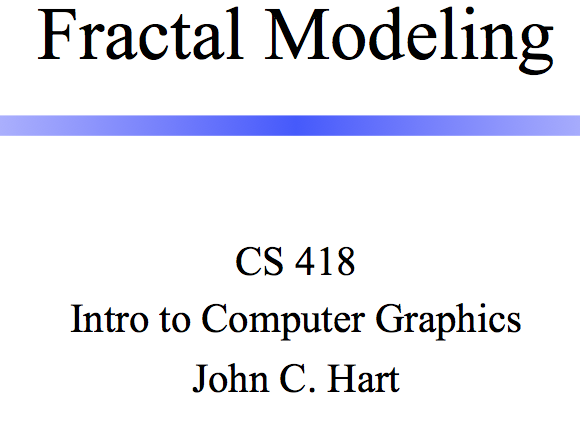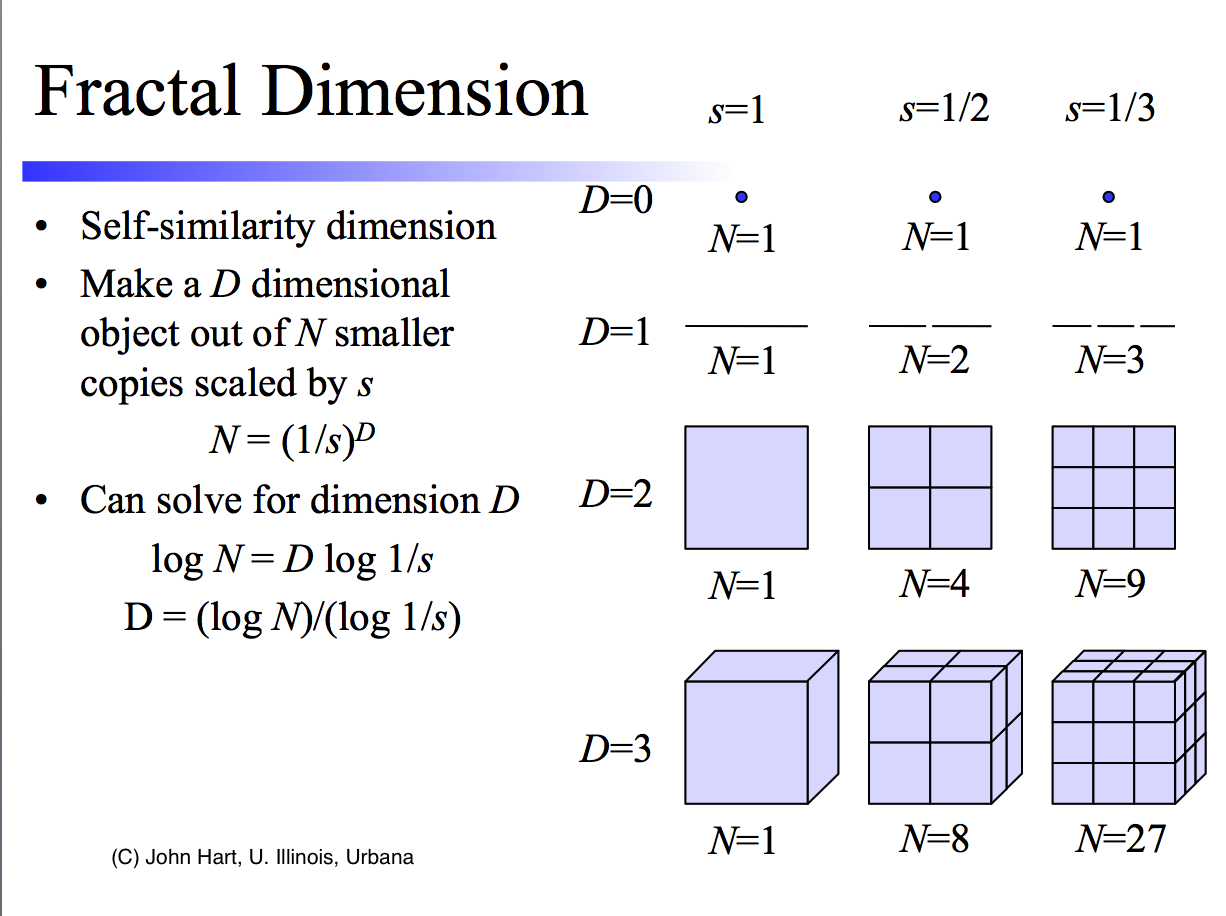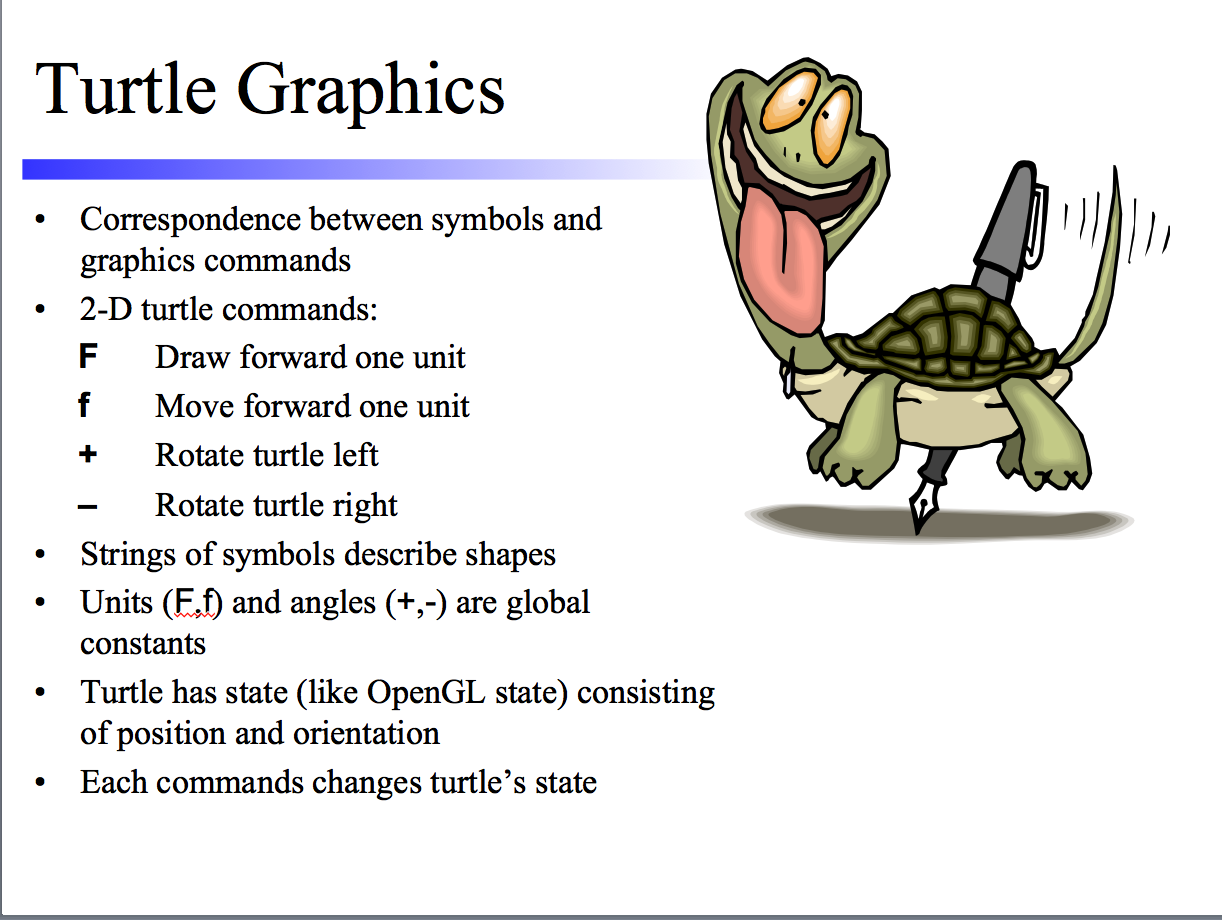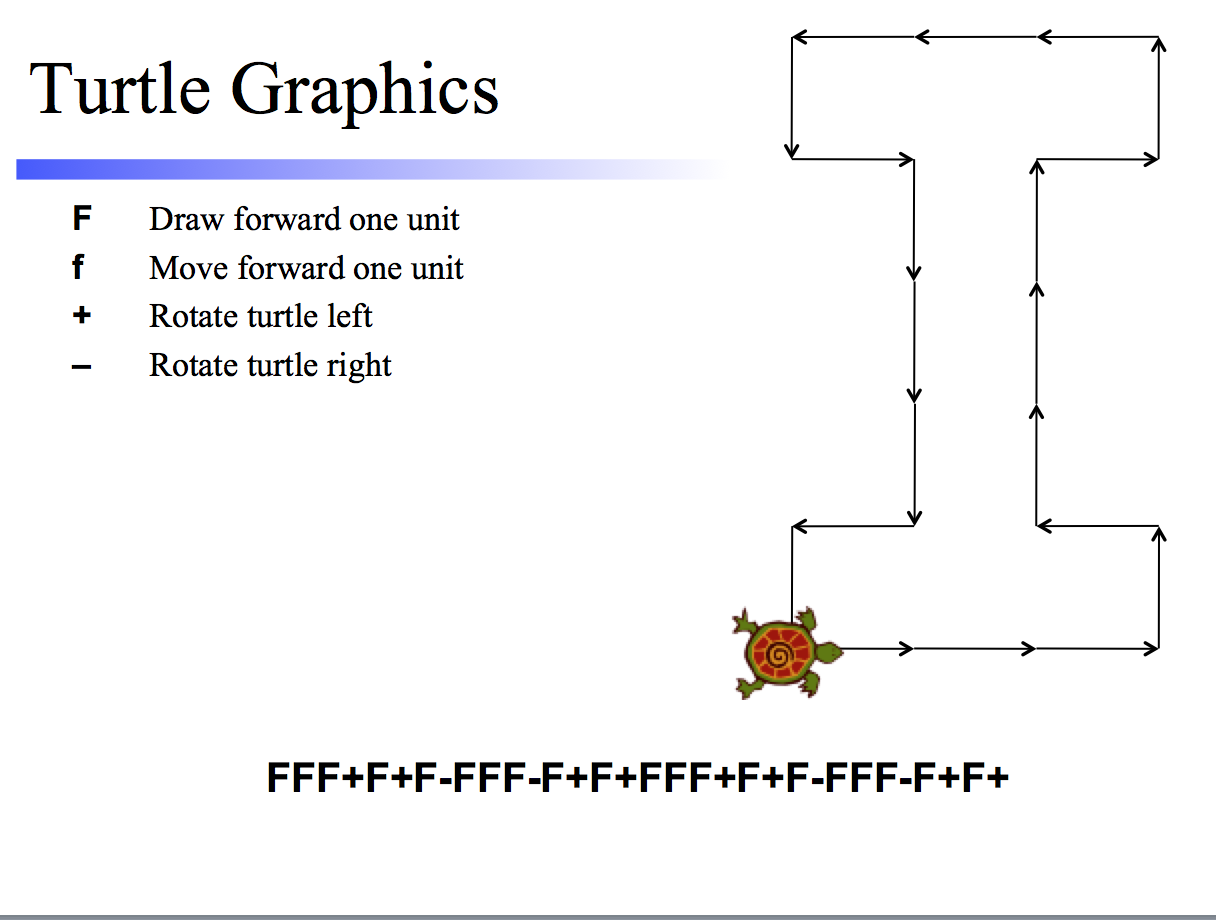On John Hart's Slides, F4 2016 "
Last edited 18sep16

\begin{document}
\maketitleSome comments on the slides shown in class F4.
\section{Fractal Dimension}Here is another way of giving a plausible, heuristic definition of the
\textit{dimension} of a self-similar geometrical figure.
The precise mathematical, or computational definitions are much more
complicated. It is the one used by Michael Barnsley in his definitive
text book on fractals.

Thus we all agree that whatever dimensions means, the point has dimension 0,
a line segment has dimensiona 1, a square 2, and a cube 3. Now consider
the quantity of each of these figures: length, area, volume respectively.

If we double the side-length of line we just double its length.
But the square on double the side-length has quadruples the area.
For cube on double the side-length, the volume is 27, or $2^3$.

If we coin the symbol $Q(F)$ for the quantity of a figure, and
write $2F$ for same figure based on twice the side-length, then we see that
$Q(2F) = 2^D Q(F)$
The number 2 is scaling factor $s$  Hart takes all the way to 3.
Taking logarithms, we can solve for the dimension
$D = \frac{log Q(sF)}{log Q(F)}$.
Note, the fractions shows that which logarithm you take doesn't matter.

\section{Koch Snowflake}This fractal is a curve defined in the plane. So stages can be drawn
using Turtle Graphics.

[To be completed later.]
\section{Sierpinski Triangle}
This fractal is a region in the plane. Its stages are obtained by removing
the inscribe center triangle from each remaining triangle.

[To be completed later.]
\section{Lindenmeyer Systems}
This is an algebraic language for describing programs that produce self-similar
fractals on a computer
[To be completed later.]\end{document}